SSC (English Medium) Class 8Maharashtra State Board
Share

# Balbharati solutions for Class 8 Mathematics chapter 17 - Circle : Chord and Arc

#### Balbharati Balbharati Class 8 Mathematics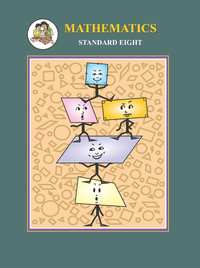## Chapter 17: Circle : Chord and Arc

Practice Set 17.1Practice Set 17.2

#### Chapter 17: Circle : Chord and Arc Exercise Practice Set 17.1 solutions [Page 116]

Practice Set 17.1 | Q 1 | Page 116

In a circle with centre P, chord AB is drawn of length 13 cm, seg PQ ⊥ chord AB, then find l(QB).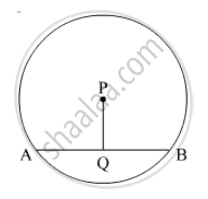Practice Set 17.1 | Q 2 | Page 116

Radius of a circle with centre O is 25 cm. Find the distance of a chord from the centre if length of the chord is 48 cm.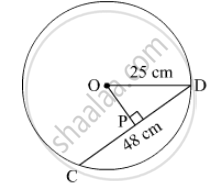Practice Set 17.1 | Q 3 | Page 116

O is centre of the circle. Find the length of radius, if the chord of length 24 cm is at a distance of 9 cm from the centre of the circle.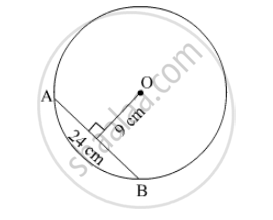Practice Set 17.1 | Q 4 | Page 116

C is the centre of the circle whose radius is 10 cm. Find the distance of the chord from the centre if the length of the chord is 12 cm.

#### Chapter 17: Circle : Chord and Arc Exercise Practice Set 17.2 solutions [Page 118]

Practice Set 17.2 | Q 1 | Page 118

The diameters PQ and RS of the circle with centre C are perpendicular to each other at C. state, why arc PS and arc SQ are congruent. Write the other arcs which are congruent to arc PS.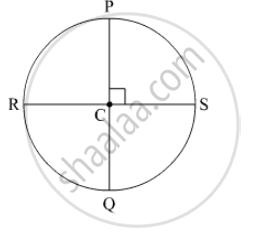Practice Set 17.2 | Q 2 | Page 118

In the adjoining figure O is the centre of the circle whose diameter is MN. Measures of some central angles are given in the figure. hence find the following.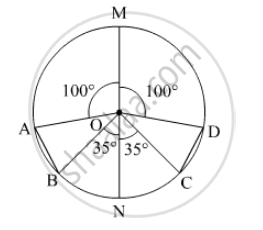(1) m∠ AOB and m∠ COD
(2) Show that arc AB ≌ arc CD.
(3) Show that chord AB ≌ chord CD

## Chapter 17: Circle : Chord and Arc

Practice Set 17.1Practice Set 17.2

#### Balbharati Balbharati Class 8 Mathematics## Balbharati solutions for Class 8 Mathematics chapter 17 - Circle : Chord and Arc

Balbharati solutions for Class 8 chapter 17 (Circle : Chord and Arc) include all questions with solution and detail explanation. This will clear students doubts about any question and improve application skills while preparing for board exams. The detailed, step-by-step solutions will help you understand the concepts better and clear your confusions, if any. Shaalaa.com has the Maharashtra State Board Balbharati Class 8 Mathematics solutions in a manner that help students grasp basic concepts better and faster.

Further, we at Shaalaa.com provide such solutions so that students can prepare for written exams. Balbharati textbook solutions can be a core help for self-study and acts as a perfect self-help guidance for students.

Concepts covered in Class 8 Mathematics chapter 17 Circle : Chord and Arc are Properties of Chord of a Circle, Arcs Corresponding to the Chord of a Circle.

Using Balbharati Class 8 solutions Circle : Chord and Arc exercise by students are an easy way to prepare for the exams, as they involve solutions arranged chapter-wise also page wise. The questions involved in Balbharati Solutions are important questions that can be asked in the final exam. Maximum students of Maharashtra State Board Class 8 prefer Balbharati Textbook Solutions to score more in exam.

Get the free view of chapter 17 Circle : Chord and Arc Class 8 extra questions for and can use Shaalaa.com to keep it handy for your exam preparation

S